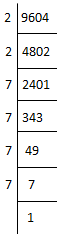# Find the square root of 9604 by prime factorization

Prime factorisation is a method to find the prime factors of a given number, say a composite number. These factors are nothing but the prime numbers. A prime number is a number which has only two factors, i.e. 1 and the number itself.

## Find the square root of 9604 by the prime factorisation method

We have to find the square root of 9604 by the prime factorisation method9604 = 2 × 2 × 7 × 7 × 7 × 7× 1

9604 = (2 × 2) × (7 × 7) × (7 × 7)

9604 = (2 × 7 × 7) × (2 × 7 ×7)

9604 = (2 × 7 × 7)2

Therefore, √9604 = 2 × 7 × 7 = 98.

## Video Lesson### Articles to Explore:(94)(36)